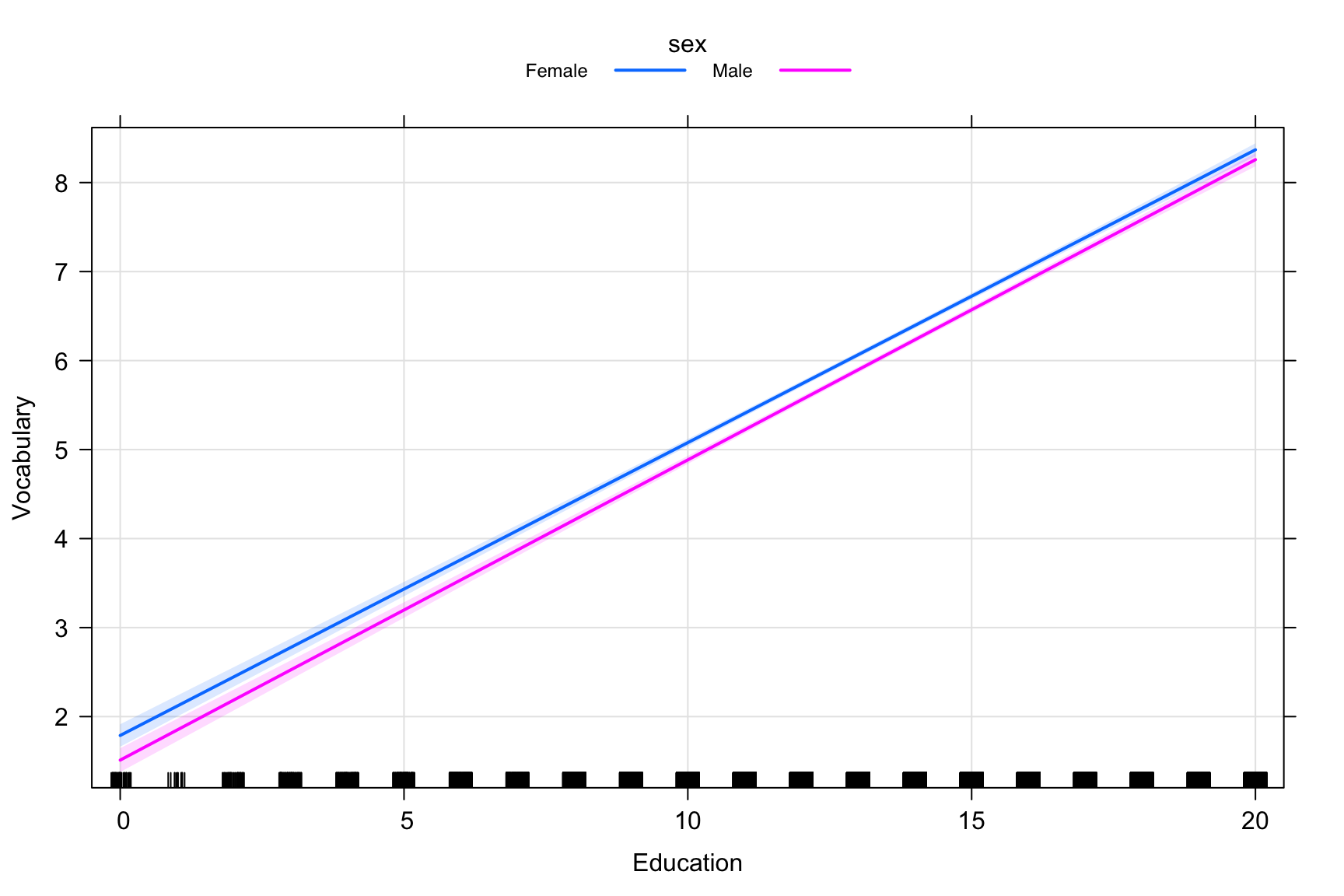# 1 Lab preparation

## 1.1$$\color{purple}{\text{Creating a version-controlled R-Project with Github}}$$

Download repository here: $$\color{blue}{\text{https://github.com/garberadamc/SEM-Lab3}}$$

On the Github repository webpage:

1. fork your own branch of the lab repository
2. copy the repository web URL address from the clone or download menu

Within R-Studio:

1. click “NEW PROJECT” (upper right corner of window)
2. choose option Version Control
3. choose option Git
4. paste the repository web URL path copied from the clone or download menu on Github page
5. choose location of the R-Project ($$\color{red}{\text{too many nested folders will result in filepath error}}$$)

## 1.2 Data source for example 1:

The first example utilizes the Vocabulary and Education dataset from the National Opinion Research Center General Social Survey. GSS Cumulative Datafile 1972-2016 (Fox, 2008) $$\color{blue}{\text{See documentation here}}$$

This dataset is available via the R-package {carData} and can be directly loaded into the R environment.

Note: All models specified in the following exercise are for demonstration only and are not theoretically justified or valid.

# equatiomatic is not yet on CRAN. Install the development version from GitHub with
remotes::install_github("datalorax/equatiomatic", force = TRUE)
library(tidyverse)
library(MplusAutomation)
library(rhdf5)
library(here)
library(gt)
library(gtsummary)
library(estimatr)
library(kableExtra)
library(hrbrthemes)
library(equatiomatic)
library(effects)
library(carData)
library(Ecdat)
library(huxtable)
library(flair)

# 2 Begin lab 2 exercise

Read the dataframe into your R-environment from package {carData}

data(Vocab)

vocab <- as.data.frame(Vocab)

Take a look at focal variables, make a tribble table
Name Labels
year Year of the survey
sex Sex of the respondent (Female or Male)
education Education, in years
vocabulary Vocabulary test score: number correct on a 10-word test

check some basic descriptives with the {gtsummary} package

table1 <- tbl_summary(vocab,
statistic = list(all_continuous() ~ "{mean} ({sd})"),
missing = "no" ) %>%
bold_labels()

table1
Characteristic N = 303511
year 1995 (13)
sex
Female 17148 (56%)
Male 13203 (44%)
education 13.0 (3.0)
vocabulary 6.00 (2.12)

1 Statistics presented: mean (SD); n (%)

Run a regression of the model with interaction of sex and education using the lm function

m1_interact <- lm(formula =                           vocabulary ~ sex +                                  education +                              sex:education ,                   data=vocab)

Print summary of regression output using package {huxtable}

huxreg(m1_interact, error_pos = 'right')
 (1) (Intercept) 1.788 *** (0.064) sexMale -0.277 ** (0.094) education 0.329 *** (0.005) sexMale:education 0.008 (0.007) N 30351 R2 0.231 logLik -61825.557 AIC 123661.113 *** p < 0.001; ** p < 0.01; * p < 0.05.

Print the Latex syntax for the regression equation using the package {equatiomatic}

extract_eq(m1_interact)

$\color{#FF851B}{\text{vocabulary}} = \alpha + \beta_{1}(\color{#0074D9}{\text{sex}_{\text{Male}}}) + \beta_{2}(\color{#2ECC40}{\text{education}}) + \beta_{3}(\color{#0074D9}{\text{sex}_{\text{Male}}} \times \color{#2ECC40}{\text{education}}) + \epsilon$

extract_eq(m1_interact, use_coefs = TRUE)

$\color{#FF851B}{\text{vocabulary}} = 1.79 - 0.28(\color{#0074D9}{\text{sex}_{\text{Male}}}) + 0.33(\color{#2ECC40}{\text{education}}) + 0.01(\color{#0074D9}{\text{sex}_{\text{Male}}} \times \color{#2ECC40}{\text{education}}) + \epsilon$

Plot the interaction effect using the package {effects}

int <- effect("sex:education",m1_interact)

plot(int, main="", grid=TRUE,
x.var = "education", xlab="Education",
ylab="Vocabulary", multiline=TRUE, confint=list(style="auto"))Figure. Example 1 interaction is non-significant (no moderation)

Filter year to include 1974 and 2016 (emphasizing moderation effect)

vocab2 <-  vocab %>%
filter(year %in% c(1974, 2016)) %>%
mutate(year = droplevels(factor(year)))

Run regression with interaction between year and education

# below is R-code to center covariate 'education'# vocab2 <- vocab %>% #   mutate(education = scale(education, scale = FALSE)) %>% #   mutate(year = scale(year, scale = FALSE))m2_interact <- lm(formula =                           vocabulary ~ sex +                                  education +                                       year +                             year:education ,                   data=vocab2)huxreg(m2_interact, error_pos = 'right')
 (1) (Intercept) 1.600 *** (0.184) sexMale -0.073 (0.062) education 0.375 *** (0.015) year2016 0.373 (0.272) education:year2016 -0.078 *** (0.021) N 3307 R2 0.244 logLik -6607.022 AIC 13226.043 *** p < 0.001; ** p < 0.01; * p < 0.05.

Print the Latex syntax for the regression equation using the package {equatiomatic}

extract_eq(m2_interact)

$\color{#FF851B}{\text{vocabulary}} = \alpha + \beta_{1}(\color{#0074D9}{\text{sex}_{\text{Male}}}) + \beta_{2}(\color{#2ECC40}{\text{education}}) + \beta_{3}(\color{#39CCCC}{\text{year}_{\text{2016}}}) + \beta_{4}(\color{#2ECC40}{\text{education}} \times \color{#39CCCC}{\text{year}_{\text{2016}}}) + \epsilon$

extract_eq(m2_interact, use_coefs = TRUE)

$\color{#FF851B}{\text{vocabulary}} = 1.6 - 0.07(\color{#0074D9}{\text{sex}_{\text{Male}}}) + 0.38(\color{#2ECC40}{\text{education}}) + 0.37(\color{#39CCCC}{\text{year}_{\text{2016}}}) - 0.08(\color{#2ECC40}{\text{education}} \times \color{#39CCCC}{\text{year}_{\text{2016}}}) + \epsilon$

Plot the interaction effect using the package {effects}

int2 <- effect("education:year", m2_interact)

plot(int2, main="", grid=TRUE,
x.var = "education", xlab="Education",
ylab="Vocabulary",
multiline=TRUE,
confint=list(style="auto"))